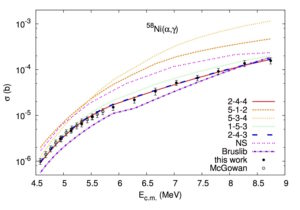Understanding the p-process requires not only information on the stellar environment in which the process takes place, but also input from nuclear physics. The latter includes nuclear masses, half-lives of the unstable nuclei and rates of all the reactions involved. The p-process is a network of (γ,p), (γ,α) and (γ,n) reactions and their inverse on about 2,000 elements present in the environment (i.e., about 20,000 reaction). Cross sections for such a large amount of reactions have to rely on models, the most popular being HF statistical model, as the p-process involves heavy nuclei. In such case, the level densities are sufficiently high and the reactions can be described by averaged quantities. The statistical model calculations require three main components as an input: level density (LD),γ-strength function (γSF) and optical model potential (OMP).